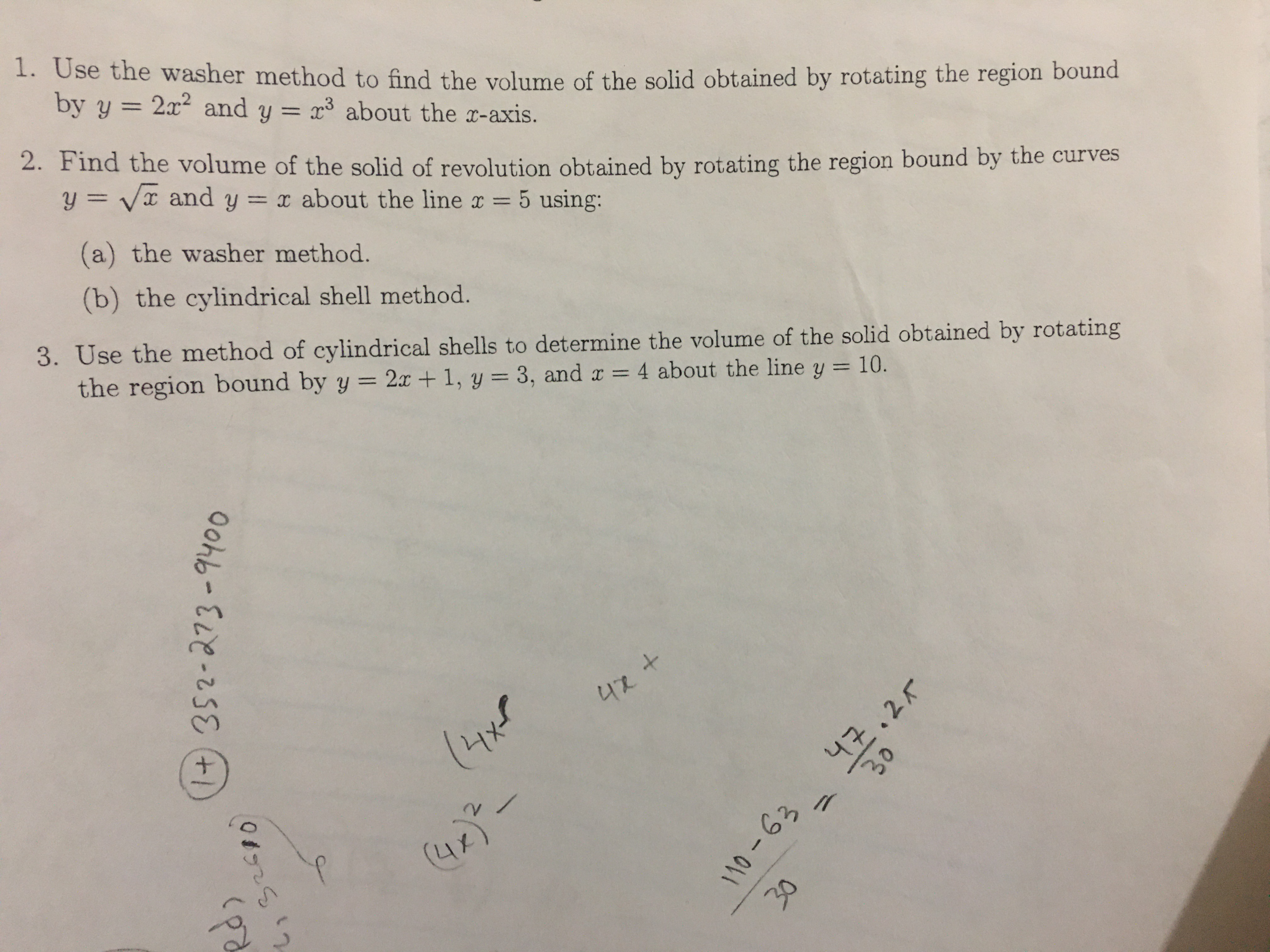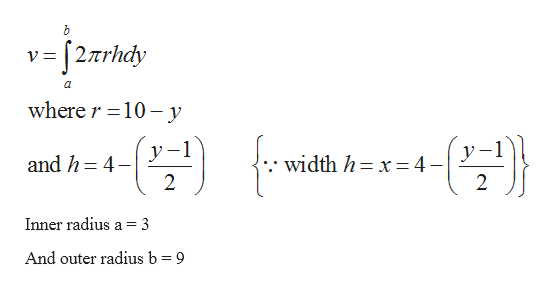# 1+ 352-273-9400L,92510110-6330301. Use the washer method to find the volume of the solid obtained by rotating the region boundby y 2x2 and y =11r about the x-axis.2. Find the volume of the solid of revolution obtained by rotating the region bound by the cuy = Vx and y= x about the line x =5 using:(a) the washer method.(b) the cylindrical shell method.3. Use the method of cylindrical shells to determine the volume of the solid obtained by rotatingthe region bound by y = 2x + 1, y = 3, and x 4 about the line y = 10.42 +(Hxf니구.2rV(4/

Question
36 views

For number 3, how to find radius and height? And how do I know for height which function to subtract?help_outlineImage Transcriptionclose1+ 352-273-9400 L,92510 110-63 30 30 1. Use the washer method to find the volume of the solid obtained by rotating the region bound by y 2x2 and y = 11 r about the x-axis. 2. Find the volume of the solid of revolution obtained by rotating the region bound by the cu y = Vx and y= x about the line x =5 using: (a) the washer method. (b) the cylindrical shell method. 3. Use the method of cylindrical shells to determine the volume of the solid obtained by rotating the region bound by y = 2x + 1, y = 3, and x 4 about the line y = 10. 42 + (Hxf 니구.2r V (4/ fullscreen
check_circle

Step 1

Given that

Given that

Step 2

To find out volume bounded by given region and lines

Step 3

Volume is giv...help_outlineImage Transcriptionclosev 27rhdy wherer 10-y -1 у- y and h 4 width h x4- 2 2 Inner radius a = 3 And outer radius b = 9 fullscreen

### Want to see the full answer?

See Solution

#### Want to see this answer and more?

Solutions are written by subject experts who are available 24/7. Questions are typically answered within 1 hour.*

See Solution
*Response times may vary by subject and question.
Tagged in

### Integration